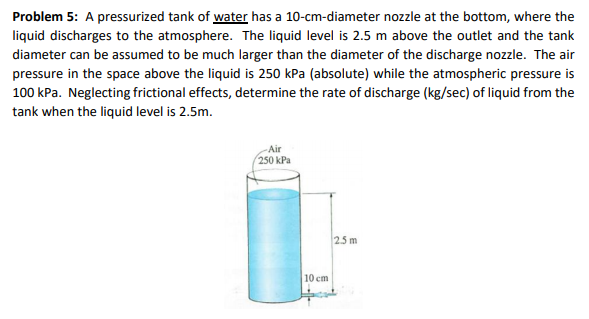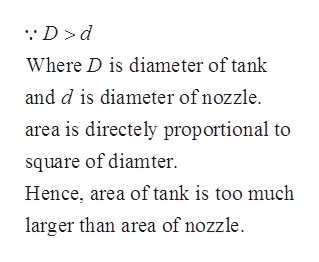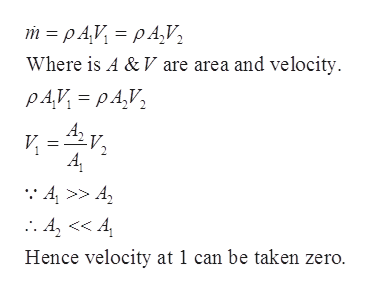# Problem 5: A pressurized tank of water has a 10-cm-diameter nozzle at the bottom, where theliquid discharges to the atmosphere. The liquid level is 2.5 m above the outlet and the tankdiameter can be assumed to be much larger than the diameter of the discharge nozzle. The airpressure in the space above the liquid is 250 kPa (absolute) while the atmospheric pressure is100 kPa. Neglecting frictional effects, determine the rate of discharge (kg/sec) of liquid from thetank when the liquid level is 2.5m.Air250 kPa25 m10 cm

Question
27 views

1. What approximations, if any, can you make from the statement that the diameter of the tank is much larger than the diameter of the nozzle?

2. Do you now have a good plan for how you will proceed with the solution of this problem?help_outlineImage TranscriptioncloseProblem 5: A pressurized tank of water has a 10-cm-diameter nozzle at the bottom, where the liquid discharges to the atmosphere. The liquid level is 2.5 m above the outlet and the tank diameter can be assumed to be much larger than the diameter of the discharge nozzle. The air pressure in the space above the liquid is 250 kPa (absolute) while the atmospheric pressure is 100 kPa. Neglecting frictional effects, determine the rate of discharge (kg/sec) of liquid from the tank when the liquid level is 2.5m. Air 250 kPa 25 m 10 cm fullscreen
check_circle

Step 1

From the given problem it has been stated that diameter of tank is larger than diameter of nozzle. This means that area of tank is too much larger than the area of nozzle.help_outlineImage Transcriptionclose:D>d Where D is diameter of tank and d is diameter of nozzle. area is directely proportional to square of diamter Hence, area of tank is too much larger than area of nozzle fullscreen
Step 2

From conservation of mass principle,

Mass flow rate will...help_outlineImage Transcriptionclosem pAV pAV Where is A &V are area and velocity PAV pA,V Av :4>> A Hence velocity at 1 can be taken zero. fullscreen

### Want to see the full answer?

See Solution

#### Want to see this answer and more?

Solutions are written by subject experts who are available 24/7. Questions are typically answered within 1 hour.*

See Solution
*Response times may vary by subject and question.
Tagged in

### Mechanical Engineering